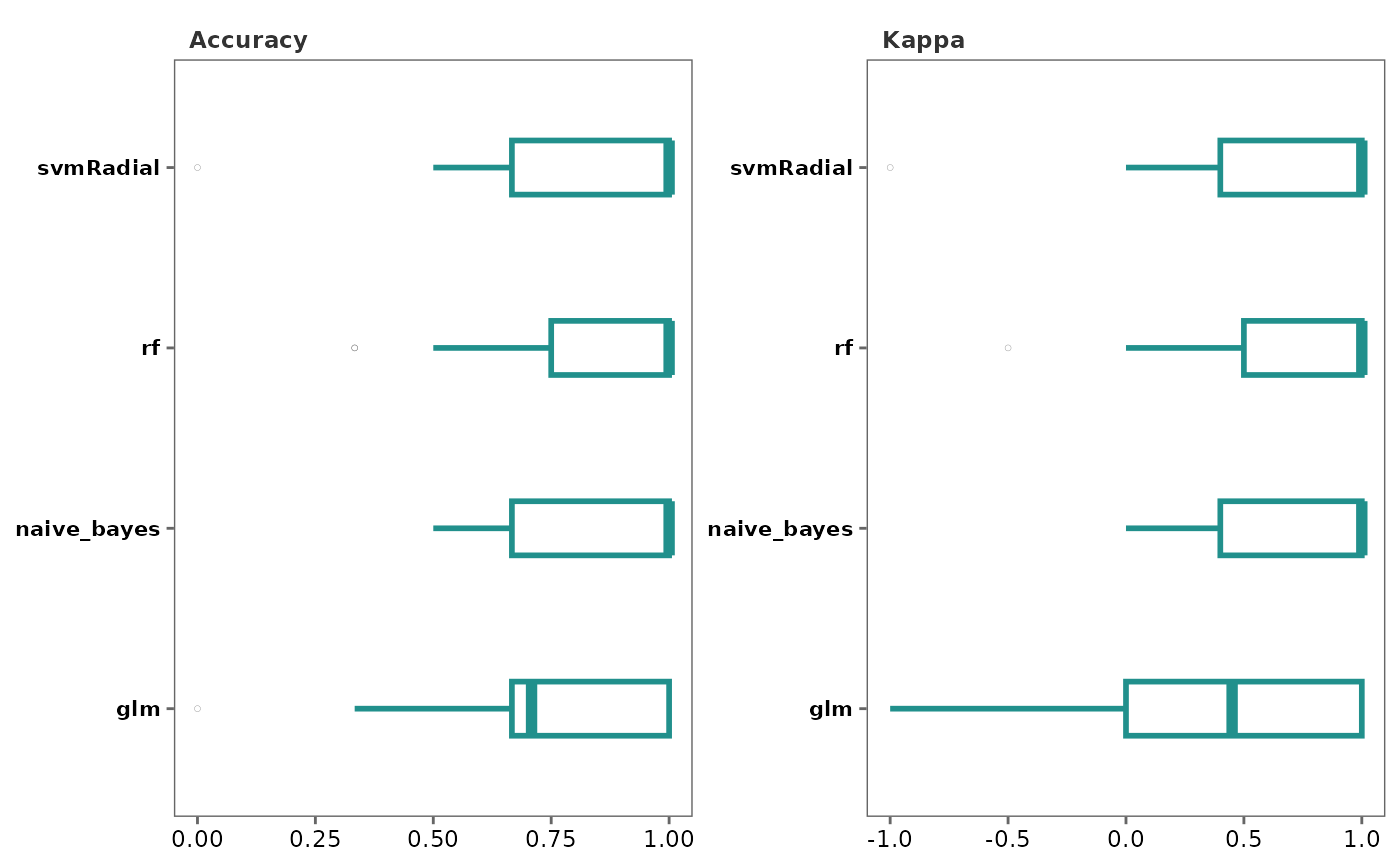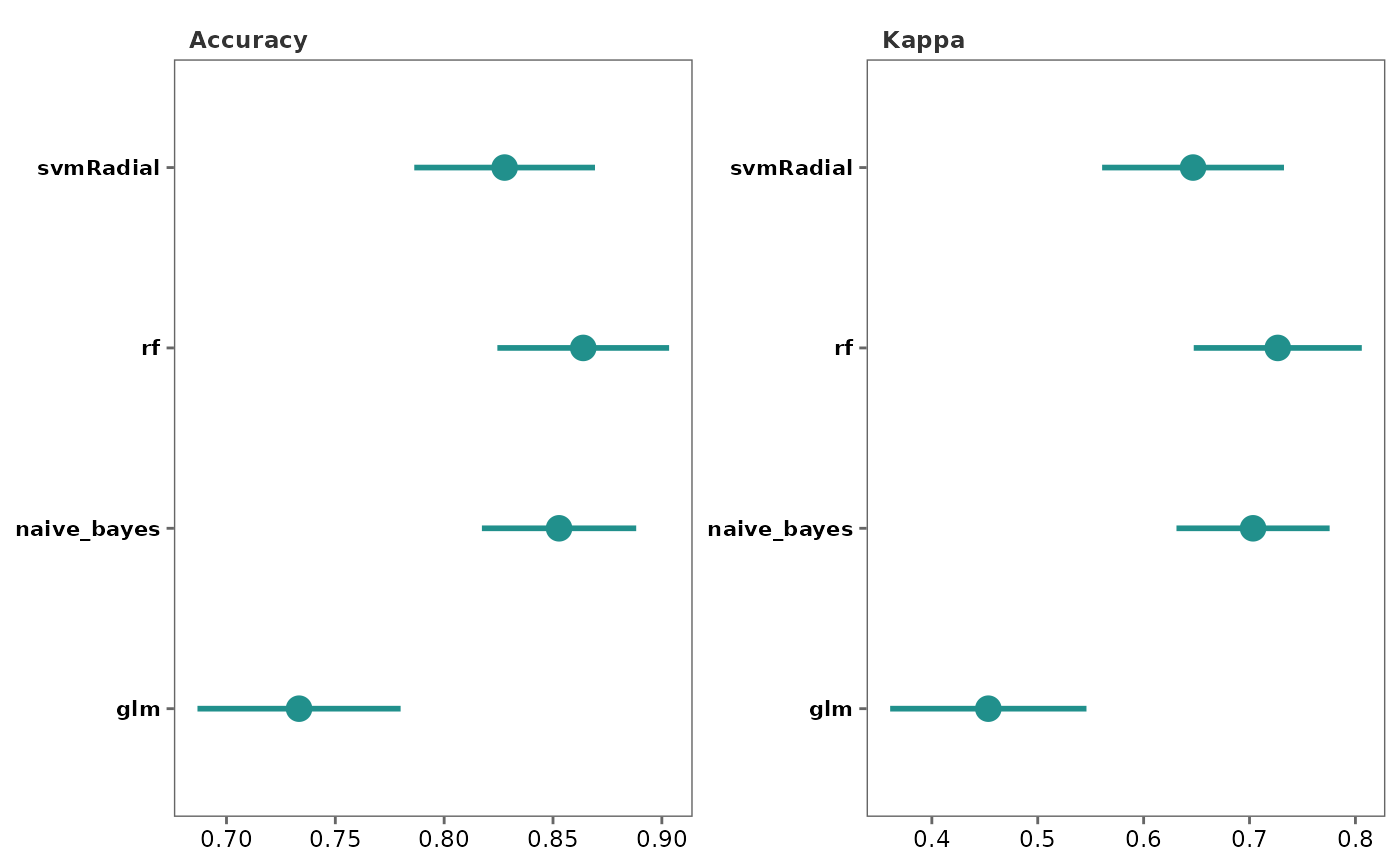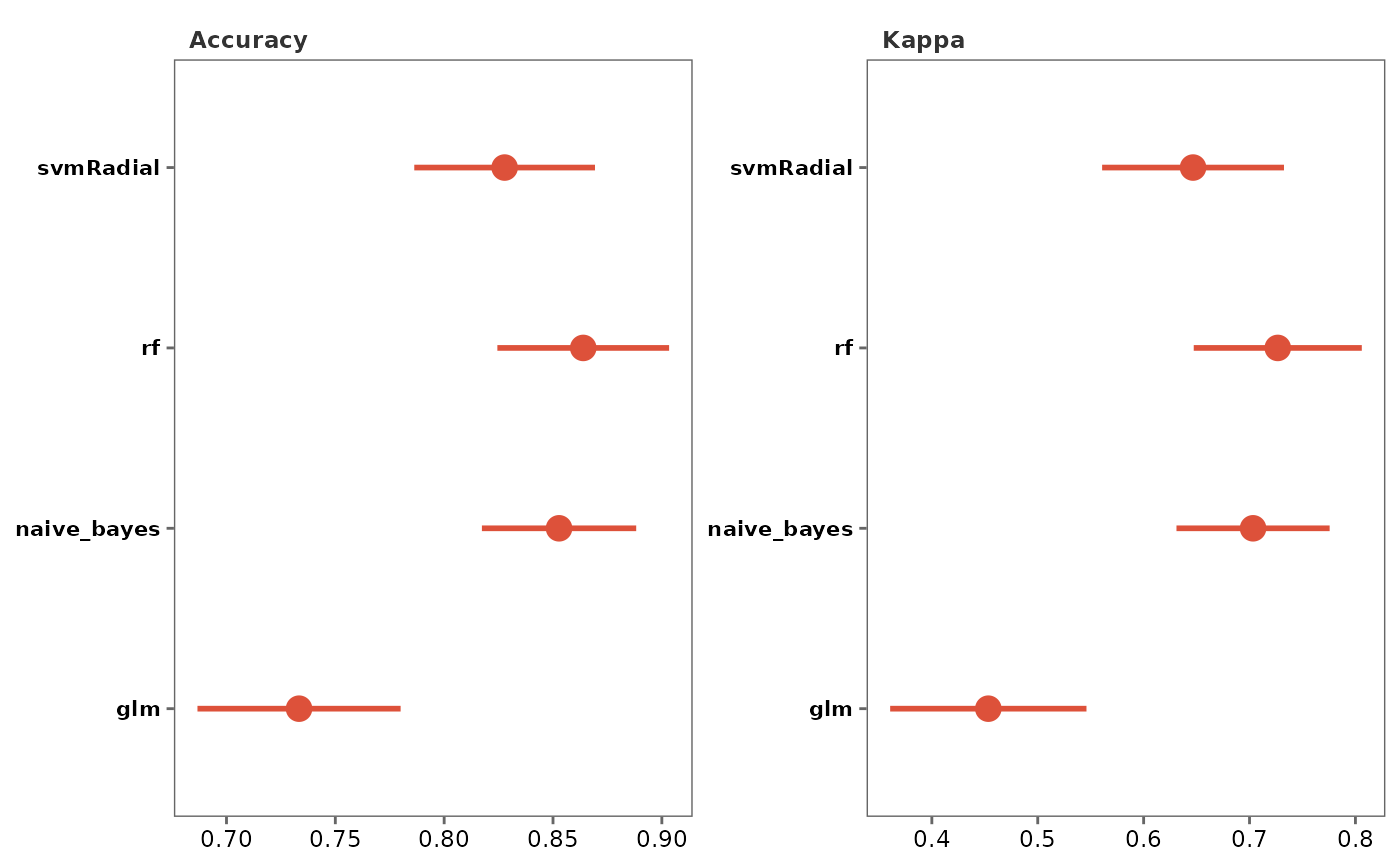This function generates plots to visualize model performance

## Usage

performance_plot(
model_list,
type = "box",
text_size = 10,
palette = "viridis",
save = FALSE,
file_path = NULL,
file_name = "Performance_plot",
file_type = "pdf",
plot_width = 7,
plot_height = 7,
dpi = 80
)

## Arguments

model_list

A model_list object from performing train_models.

type

Type of plot to generate. Choices are "box" or "dot." Default is "box." for boxplots.

text_size

Text size for plot labels, axis labels etc. Default is 10.

palette

Viridis color palette option for plots. Default is "viridis". See viridis for available options.

save

Logical. If TRUE saves a copy of the plot in the directory provided in file_path.

file_path

A string containing the directory path to save the file.

file_name

File name to save the plot. Default is "Performance_plot."

file_type

File type to save the plot. Default is "pdf".

plot_width

Width of the plot. Default is 7.

plot_height

Height of the plot. Default is 7.

dpi

Plot resolution. Default is 80.

## Value

A ggplot2 object.

## Details

• The default metrics used for classification based models are "Accuracy" and "Kappa."

• These metric types can be changed by providing additional arguments to the train_models function. See train and trainControl for more information.

• train_models

• resamples

• train

• trainControl

## Author

Chathurani Ranathunge

## Examples

# \donttest{

## Create a model_df object
covid_model_df <- pre_process(covid_fit_df, covid_norm_df)
#> Total number of differentially expressed proteins (8) is less than n_top.
#> None of the proteins show high pair-wise correlation.
#>
#> No highly correlated proteins to be removed.

## Split the data frame into training and test data sets
covid_split_df <- split_data(covid_model_df)

## Fit models based on the default list of machine learning (ML) algorithms
covid_model_list <- train_models(covid_split_df)
#>
#>
#> Running rf...
#>
#> Running glm...
#> Warning: glm.fit: algorithm did not converge
#> Warning: glm.fit: fitted probabilities numerically 0 or 1 occurred
#> Warning: glm.fit: algorithm did not converge
#> Warning: glm.fit: fitted probabilities numerically 0 or 1 occurred
#> Warning: glm.fit: algorithm did not converge
#> Warning: glm.fit: fitted probabilities numerically 0 or 1 occurred
#> Warning: glm.fit: algorithm did not converge
#> Warning: glm.fit: fitted probabilities numerically 0 or 1 occurred
#> Warning: glm.fit: algorithm did not converge
#> Warning: glm.fit: fitted probabilities numerically 0 or 1 occurred
#> Warning: glm.fit: algorithm did not converge
#> Warning: glm.fit: fitted probabilities numerically 0 or 1 occurred
#> Warning: glm.fit: algorithm did not converge
#> Warning: glm.fit: fitted probabilities numerically 0 or 1 occurred
#> Warning: glm.fit: algorithm did not converge
#> Warning: glm.fit: fitted probabilities numerically 0 or 1 occurred
#> Warning: glm.fit: algorithm did not converge
#> Warning: glm.fit: fitted probabilities numerically 0 or 1 occurred
#> Warning: glm.fit: algorithm did not converge
#> Warning: glm.fit: fitted probabilities numerically 0 or 1 occurred
#> Warning: glm.fit: algorithm did not converge
#> Warning: glm.fit: fitted probabilities numerically 0 or 1 occurred
#> Warning: glm.fit: algorithm did not converge
#> Warning: glm.fit: fitted probabilities numerically 0 or 1 occurred
#> Warning: glm.fit: algorithm did not converge
#> Warning: glm.fit: fitted probabilities numerically 0 or 1 occurred
#> Warning: glm.fit: algorithm did not converge
#> Warning: glm.fit: fitted probabilities numerically 0 or 1 occurred
#> Warning: glm.fit: algorithm did not converge
#> Warning: glm.fit: fitted probabilities numerically 0 or 1 occurred
#> Warning: glm.fit: algorithm did not converge
#> Warning: glm.fit: fitted probabilities numerically 0 or 1 occurred
#> Warning: glm.fit: algorithm did not converge
#> Warning: glm.fit: fitted probabilities numerically 0 or 1 occurred
#> Warning: glm.fit: algorithm did not converge
#> Warning: glm.fit: fitted probabilities numerically 0 or 1 occurred
#> Warning: glm.fit: algorithm did not converge
#> Warning: glm.fit: fitted probabilities numerically 0 or 1 occurred
#> Warning: glm.fit: algorithm did not converge
#> Warning: glm.fit: fitted probabilities numerically 0 or 1 occurred
#> Warning: glm.fit: algorithm did not converge
#> Warning: glm.fit: fitted probabilities numerically 0 or 1 occurred
#> Warning: glm.fit: algorithm did not converge
#> Warning: glm.fit: fitted probabilities numerically 0 or 1 occurred
#> Warning: glm.fit: algorithm did not converge
#> Warning: glm.fit: fitted probabilities numerically 0 or 1 occurred
#> Warning: glm.fit: algorithm did not converge
#> Warning: glm.fit: fitted probabilities numerically 0 or 1 occurred
#> Warning: glm.fit: algorithm did not converge
#> Warning: glm.fit: fitted probabilities numerically 0 or 1 occurred
#> Warning: glm.fit: algorithm did not converge
#> Warning: glm.fit: fitted probabilities numerically 0 or 1 occurred
#> Warning: glm.fit: algorithm did not converge
#> Warning: glm.fit: fitted probabilities numerically 0 or 1 occurred
#> Warning: glm.fit: algorithm did not converge
#> Warning: glm.fit: fitted probabilities numerically 0 or 1 occurred
#> Warning: glm.fit: algorithm did not converge
#> Warning: glm.fit: fitted probabilities numerically 0 or 1 occurred
#> Warning: glm.fit: algorithm did not converge
#> Warning: glm.fit: fitted probabilities numerically 0 or 1 occurred
#> Warning: glm.fit: algorithm did not converge
#> Warning: glm.fit: fitted probabilities numerically 0 or 1 occurred
#>
#> Running xgbLinear...
#>
#> Running naive_bayes...
#> Done!

## Generate box plots to visualize performance of different ML algorithms
performance_plot(covid_model_list)
#> Using Resample as id variables## Generate dot plots
performance_plot(covid_model_list, type = "dot")
#> Using Resample as id variables
#> Warning: Removed 5 rows containing missing values (geom_segment()).
#> Warning: Removed 5 rows containing missing values (geom_segment()).## Change color palette
performance_plot(covid_model_list, type = "dot", palette = "inferno")
#> Using Resample as id variables
#> Warning: Removed 5 rows containing missing values (geom_segment()).
#> Warning: Removed 5 rows containing missing values (geom_segment()).# }